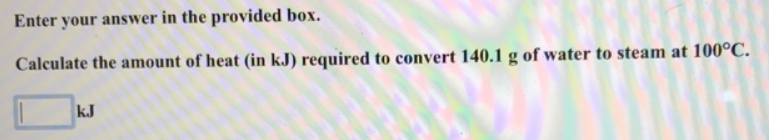Chemistry Practice Problems Heating and Cooling Curves Practice Problems Solution: Enter your answer in the provided box.Calculate th...

# Solution: Enter your answer in the provided box.Calculate the amount of heat (in kJ) required to convert 140.1 g of water to steam at 100 °C.

###### Problem

Calculate the amount of heat (in kJ) required to convert 140.1 g of water to steam at 100 °C.View Complete Written Solution

Heating and Cooling Curves

Heating and Cooling Curves

#### Q. How much heat energy is required to convert 97.9 g of liquid sulfur dioxide, SO2, at 202.6 K to gaseous SO2 at 263.1 K if the molar heat of vaporizati...

Solved • Wed Aug 08 2018 14:14:05 GMT-0400 (EDT)

Heating and Cooling Curves

#### Q. How many grams of benzene, C6H6, can be melted with 39.7 kJ of heat energy? The molar heat of fusion of benzene is 9.95 kJ/mol.

Solved • Wed Aug 08 2018 14:12:16 GMT-0400 (EDT)

Heating and Cooling Curves

#### Q. How much heat energy is required to melt 388.9 g of HBr? The molar heat of fusion of HBr is 2.41 kJ/mol.

Solved • Wed Aug 08 2018 14:07:05 GMT-0400 (EDT)

Heating and Cooling Curves

#### Q. How much heat energy is required to boil 93.7 g of ammonia, NH3? The molar heat of vaporization of ammonia is 23.4 kJ/mol.

Solved • Wed Aug 08 2018 14:06:04 GMT-0400 (EDT)